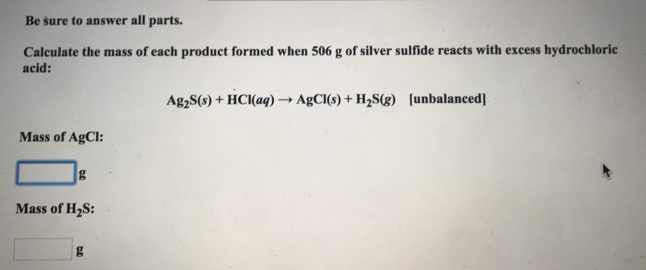# Problem: Be sure to answer all parts. Calculate the mass of each product formed when 506 g of silver sulfide reacts with excess hydrochlorie acid: Ag2S (s) + HCI (aq) → AgCI (s) + H2S (g) [unbalanced] Mass of AgCl: Mass of H2S:

###### FREE Expert Solution
90% (86 ratings)###### Problem Details

Be sure to answer all parts.

Calculate the mass of each product formed when 506 g of silver sulfide reacts with excess hydrochlorie acid:

Ag2S (s) + HCI (aq) → AgCI (s) + H2S (g) [unbalanced]

Mass of AgCl:

Mass of H2S: